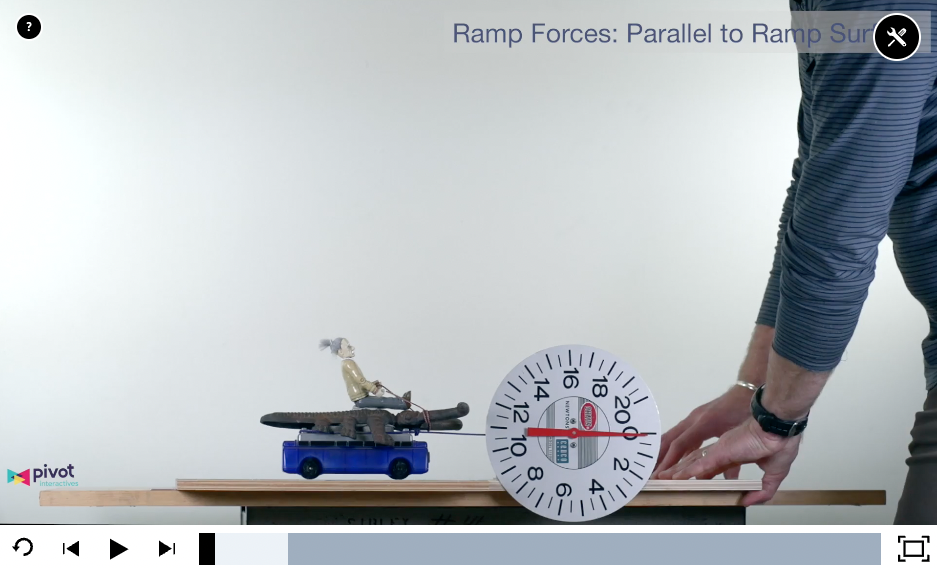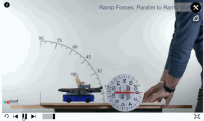# 48 minutes of Active Learning in One Class Period

September 27, 2022# 48 minutes of active learning: Data, graphing, models, direct instruction, models again!

## Peter Bohacek is a physics teacher in the Twin Cities and the co-founder of Pivot Interactives. Using forces on objects on ramps as an example, he shares how he packs many skills and concepts into a dense, active, but coherent activity in a single 48- minute class period, without extensive prep time.

### Blog Originally Written in February 2020Having the data table and graph combined with the interactive video allows students create a data table, collect data, and plot string tension vs ramp angle in just minutes.

In class on Monday, our topic was forces on object on ramps. Normally, I find that direct instruction is the fastest way to teach this, at least as an introduction. This time I tried a new approach, using Pivot Interactives, and I was thrilled with the results. We were able to pack many skills and concepts into a dense, active, but coherent activity that fit in a single 48- minute class period. Like most teachers, my available prep time is limited, so it was an added benefit that little set-up was needed for this activity. Here’s what we did:

1. Demo: a cart on a ramp, held in place by a string connected to the top of the ramp (or, even quicker, you can draw this on the board).(2 min)

2. Think-Pair-Share: Ask students what they think will happen to the string tension as the ramp angle is increased, and what will happen to the normal force (or support force) on the cart by the ramp as the ramp angle is increased. Students will readily agree that the string tension increases. But what’s the pattern for how this force changes? (5 min)

3. Students open Pivot Interactives: Forces on Objects on a Ramp (short version). We’d completed Part 1 as class discourse, so we went right to part 2. Follow instructions to collect 10 data points for the string tension as the ramp angle increases. When students have collected data, ask them to try to “linearize” the data to find the mathematical relationship between string tension and ramp angle (10-15 min). Most likely they will not find a function, since they may never have seen an example where they needed a sine function to linearize.

4. Direct Instruction: resolving the force of gravity into components. Show students how to use geometry to resolve the force of gravity into components that are aligned with axes that are tilted to match the ramp surface. The result shows that the component of the force of gravity parallel to the ramp surface is equal to mg sinθ. In addition, if the string holds an object on a ramp in equilibrium the string tension will also be equal to mg sinθ. (15 min)

5. Data: Go back to the data table and graph and try using the sine function to linearize. Add a column to the data table: sinθ and plot string tension on the vertical axis and sinθ on the horizontal axis. The fit is so beautiful and satisfying! (5 min)

6. Interpret: Ask students to determine the physical meaning of the slope of the line. Comparing the equation for the line on their graph (F=slope x sinθ) compared to the equation we got using geometry (F=mg sinθ) students will see that the slope is equal to the weight of the object. Many will realize that when θ=90°, sinθ=1 so when the ramp is vertical, the string tension is equal to the weight of the object. (3 min)

Students are now ready to apply this technique to any scenario where an object is on a ramp. But they’ll have a concrete experience to help them understand why the force of gravity parallel with the ramp is mg sinθ

What’s so nice is that this entire process can fit into one class period. Students are engaged in multiple instructional modes: peer discussion, measurement, data collection and graphing, data analysis, direct instruction, then more data analysis, and sense making.

This is a perfect example of the power of Pivot Interactives. Students can quickly use a real phenomenon and real measurements to see the connection between science content and practical applications. As always, our goal is to create ways for teachers to use real phenomena, real data to see the how science describes nature.